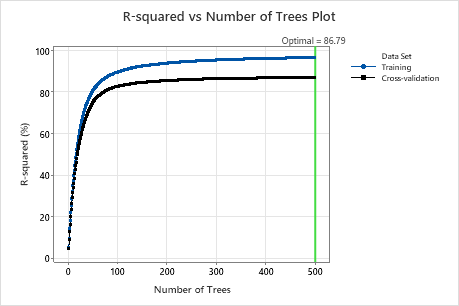# R-squared vs number of trees plot for Fit Model and Discover Key Predictors with TreeNet® Regression

###### Note

This command is available with the Predictive Analytics Module. Click here for more information about how to activate the module.

The R-squared vs Number of Trees Plot displays R2 values on the y-axis and the number of trees on the x-axis. The R2 value indicates whether the model is a good fit. Use the test results to assess the performance of the model to predict new observations. Compare the training results and the test results to see whether there are overfitting problems with the model for the training data set.This analysis grows 500 trees. The optimal number of trees is 500. The R2 value for the test data when the number of trees is 500 is approximately 86.79%.

When the absolute deviation loss function determines the number of trees for the optimal model, then Minitab displays the MAD vs Number of Trees plot.

## Interpretation

Higher values of R2 indicate a better model. The reference line indicates the optimal R2 value for the test data and the number of trees in the model. If the test curve indicates an insufficient model, consider whether to retry the analysis with alternative settings, such as larger or smaller learning rates, or a larger subsample fraction.

By using this site you agree to the use of cookies for analytics and personalized content.  Read our policy